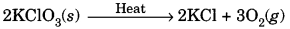# 10th Standard Science Chapter 1

10th Standard Science Chapter 1 Quiz. how much you know or you don’t  know,  test here. quiz  contains only 10 questions there no time limit.

0
Created on Bywefru

10th Standard Science Chapter 1

1 / 14

Q1) Which of the following are exothermic processes? (i) Reaction of water with quick lime
(ii) Dilution of an acid
(iii) Evaporation of water
(iv) Sublimation of camphor (crystals)

2 / 14

Question 2.
A teacher gave two test tubes to the students, one containing water and the other containing sodium hydroxide. She asked them to identify the test tube containing sodium hydroxide solution. Which one of the following can be used for the identification?

3 / 14

Question 3.
Which of the following is not physical change?

4 / 14

Question 4.
The following reaction is an example of a
4NH3(g) + 5O2(g) → 4NO(g) + 6H2O(g)
(i) displacement reaction
(ii) combination reaction
(iii) redox reaction
(iv) neutralisation reaction

5 / 14

Question 5.
Which of the following is true for an unbalanced chemical equation?

6 / 14

Question 6.
Which option denotes a double displacement reaction?

7 / 14

Question 7.
Which among the following is (are) double displacement reaction(s)?
(t) Pb + CuCl → PbCl2 + Cu
(ii) Na2SO4 + BaCl2 → BaSO4 + 2NaCl
(iii) C + O2 → CO2
(iv) CH4 + 2O2 → CO2 + 2H2O

8 / 14

Question 8.
The following reaction is used for the preparation of oxygen gas in the laboratory:Which of the following statement about the reaction is correct?

9 / 14

Question 9.
Which of the following is Not True with respect to the neutralisation reaction?

10 / 14

Question 11.
Combustion reactions are always

11 / 14

Question 12.
Barium chloride on reacting with ammonium sulphate forms barium sulphate and ammonium chloride. Which of the following correctly represents the type of the reaction involved?Displacement reaction
(ii) Precipitation reaction
(iii) Combination reaction
(iv) Double displacement reaction

12 / 14

Question 13.
Which one of the following processes involve chemical reactions?

13 / 14

Question 14.
In the double displacement reaction between aqueous potassium iodide and aqueous lead nitrate, a yellow precipitate of lead iodide is formed. While performing the activity if lead nitrate is not available, which of the following can be used in place of lead nitrate?

14 / 14

Question 15.
In which of the following chemical equations, the abbreviations represent the correct states of the reactants and products involved at reaction temperature?